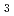The test of the ITER Central Solenoid (CS) model coil, CS and NbAl inserts was carried. The AC loss measurement is one of the most important tests to determine coil performance. The coupling losses of CS and NbAl inserts were measured by calorimetric and magnetization methods, respectively. Both coils had coupling losses with many coupling time constant, and induced circulation currents were observed by voltage taps and hall sensors. Coupling losses decreased with exponential decay constants since the electromagnetic force broke weak sinter among the strands. The gap, created between the cable and the conduit by electromagnetic force, is dependent on the pressure drop of the coolant that flows in the conductor. Our analysis shows that the exponential decay constant of coupling losses is dependent on the gap size. If we can evaluate this exponential decay constant and apply the electromagnetic force that corresponds for the exponential decay constant to the conductor before the regular operation, we can use coils of the condition that coupling losses decrease on the operation.